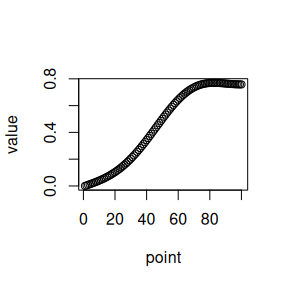# Getting started with functional statistical testing

library(funStatTest)

The funStatTest package implements various statistics for two sample comparison testing regarding functional data.

This package is developed by:

It implements statistics (and related experiments) introduced and used in .

## Data simulation

Here are some functions used to simulate clustered trajectories of functional data based on the Karhunen-Loève decomposition.

The functional data simulation process is described in  (section 3.1).

### Simulate a single trajectory

simu_vec <- simul_traj(100)
plot(simu_vec, xlab = "point", ylab = "value")### Simulate trajectories from two samples diverging by a delta

simu_data <- simul_data(
n_point = 100, n_obs1 = 50, n_obs2 = 75, c_val = 10,
delta_shape = "constant", distrib = "normal"
)
str(simu_data)
#> List of 5
#>  $mat_sample1: num [1:100, 1:50] 10 10.1 10.2 10.3 10.4 ... #>$ mat_sample2: num [1:100, 1:75] 0 0.00241 0.00497 0.00775 0.01071 ...
#>  $c_val : num 10 #>$ distrib    : chr "normal"
MatY <- simu_data$mat_sample2 stat_wmw(MatX, MatY) #>  0.9984562 ### HKR statistics The Horváth-Kokoszka-Reeder statistics  (noted $$HKR1$$ and $$HKR2$$ in ) are implemented in the stat_hkr() function. simu_data <- simul_data( n_point = 100, n_obs1 = 50, n_obs2 = 75, c_val = 10, delta_shape = "constant", distrib = "normal" ) MatX <- simu_data$mat_sample1
MatY <- simu_data$mat_sample2 stat_hkr(MatX, MatY) #>$T1
#>  56962058560
#>
#> $T2 #>  297023.2 #> #>$eigenval
#>   3.664005e+01 5.308985e+00 1.522118e+00 6.759658e-01 2.275435e-01
#>   1.705847e-01 9.892918e-02 6.026029e-02 3.071646e-02 1.788522e-02
#>  1.102164e-02 9.424057e-03 6.172824e-03 5.108395e-03 2.334137e-03
#>  1.450027e-03 3.118851e-04 6.975138e-05 1.716764e-09

### CFF statistic

The Cuevas-Febrero-Fraiman statistic  (noted $$CFF$$ in ) is implemented in the stat_cff() function.

simu_data <- simul_data(
n_point = 100, n_obs1 = 50, n_obs2 = 75, c_val = 10,
delta_shape = "constant", distrib = "normal"
)

MatX <- simu_data$mat_sample1 MatY <- simu_data$mat_sample2

stat_cff(MatX, MatY)
#>  511045.7

### Compute multiple statistics

The function comp_stat() allows to compute multiple statistics defined above in a single call on the same data.

simu_data <- simul_data(
n_point = 100, n_obs1 = 50, n_obs2 = 75, c_val = 10,
delta_shape = "constant", distrib = "normal"
)

MatX <- simu_data$mat_sample1 MatY <- simu_data$mat_sample2

res <- comp_stat(MatX, MatY, stat = c("mo", "med", "wmw", "hkr", "cff"))
res
#> $mo #>  0.9989056 #> #>$med
#>  0.9992861
#>
#> $wmw #>  0.9985099 #> #>$hkr
#>            [,1]
#> T1 5.132184e+10
#> T2 3.018523e+05
#>
#> $cff #>  511580.1 ## Permutation-based computation of p-values P-values associated to the different statistics defined above can be computed with the permutation-based method as follow: # simulate small data for the example simu_data <- simul_data( n_point = 20, n_obs1 = 4, n_obs2 = 5, c_val = 10, delta_shape = "constant", distrib = "normal" ) MatX <- simu_data$mat_sample1
MatY <- simu_data$mat_sample2 res <- permut_pval( MatX, MatY, n_perm = 100, stat = c("mo", "med", "wmw", "hkr", "cff"), verbose = TRUE) res #>$mo
#>  0.01980198
#>
#> $med #>  0.01980198 #> #>$wmw
#>  0.01980198
#>
#> $hkr #> T1 T2 #> 0.01980198 0.01980198 #> #>$cff
#>  0.01980198

:warning: computing p-values based on permutations may take some time (for large data or when using a large number of simulations. :warning:

## Simulation-based power analysis

We use our simulation scheme with permutation-based p-values computation to run a power analysis to evaluate the different statistics.

# simulate a few small data for the example
res <- power_exp(
n_simu = 20, alpha = 0.05, n_perm = 100,
stat = c("mo", "med", "wmw", "hkr", "cff"),
n_point = 25, n_obs1 = 4, n_obs2 = 5, c_val = 10, delta_shape = "constant",
distrib = "normal", max_iter = 10000, verbose = FALSE
)
res$power_res #>$mo
#>  1
#>
#> $med #>  1 #> #>$wmw
#>  1
#>
#> $hkr #> T1 T2 #> 1 1 #> #>$cff
#>  1